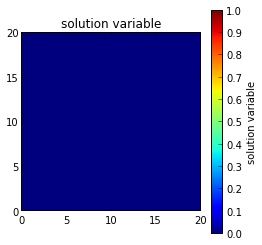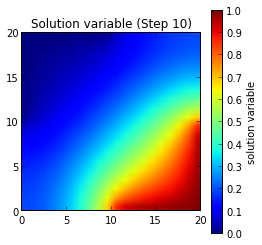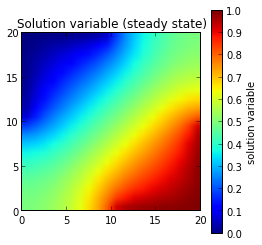# mesh20x20 FiPy example implemented as an IPython notebook¶

This examples solves a two-dimensional diffusion problem in a square domain and demonstrates the use of applying boundary condition patches.

In :
from IPython.display import clear_output
import time

In :
from fipy import *

In :
nx = 20
ny = nx
dx = 1.
dy = dx
L = dx * nx
mesh = Grid2D(dx=dx, dy=dy, nx=nx, ny=ny)


We create a CellVariable and initialize it to zero:

In :
phi = CellVariable(name = "solution variable",
mesh = mesh,
value = 0.)


and then create a diffusion equation. This is solved by default with an iterative conjugate gradient solver.

In :
D = 1.
eq = TransientTerm() == DiffusionTerm(coeff=D)


We apply Dirichlet boundary conditions

In :
valueTopLeft = 0
valueBottomRight = 1


to the top-left and bottom-right corners. Neumann boundary conditions are automatically applied to the top-right and bottom-left corners.

In :
X, Y = mesh.faceCenters
facesTopLeft = ((mesh.facesLeft & (Y > L / 2))
| (mesh.facesTop & (X < L / 2)))
facesBottomRight = ((mesh.facesRight & (Y < L / 2))
| (mesh.facesBottom & (X > L / 2)))

In :
phi.constrain(valueTopLeft, facesTopLeft)
phi.constrain(valueBottomRight, facesBottomRight)


We create a viewer to see the results

In :
viewer = Viewer(vars=phi, datamin=0., datamax=1.)and solve the equation by repeatedly looping in time:

In :
timeStepDuration = 10 * 0.9 * dx**2 / (2 * D)
steps = 10
for step in range(steps):
eq.solve(var=phi,
dt=timeStepDuration)
viewer.axes.set_title("Solution variable (Step %d)" % (step + 1,))
viewer.plot()
time.sleep(1)
clear_output()
display(viewer.axes.get_figure())We can also solve the steady-state problem directly

In :
DiffusionTerm().solve(var=phi)
viewer.plot()